﻿ Teachers’ Choice and Use of Examples in Teaching DerivativesPublications are Open
Access in this journal
Article Versions
Export Article
Cite this article
• Normal Style
• MLA Style
• APA Style
• Chicago Style
Research Article
Open Access Peer-reviewed

### Teachers’ Choice and Use of Examples in Teaching Derivatives

Chih-Hsien Huang
American Journal of Educational Research. 2017, 5(11), 1152-1157. DOI: 10.12691/education-5-11-6
Published online: November 30, 2017

### Abstract

Students’ understanding of a mathematical idea depends upon the examples used by their teachers. Thus, the key responsibility of a math teacher is to select examples that provide the best learning opportunities for students, as well as present the examples in the way most suitable to promote learning. The study reported here is part of a larger research program addressing the role and nature of examples in mathematical thinking, learning, and teaching. The main goal of this part of the study is to characterize mathematics teachers’ choice and use of examples in teaching derivatives. This study provided evidence of the application of instructional examples to teach derivatives in calculus courses.

### 1. Introduction

Students’ understanding of a mathematical idea depends upon the examples used by their teachers 1, 2. This is because the content of the examples and the methods that teachers use to guide students’ attention towards such content are correlated with students’ understanding 3. Thus, the key responsibility of a math teacher is to select examples that provide the best learning opportunities for students, as well as present the examples in the way most suitable to promote learning. Many studies have focused on the use of exemplification and examples when students learn a new mathematical concept and corresponding proofs 4, 5; however, very few studies have investigated the selection of examples by university teachers in teaching classes on differentiation and derivatives. Given that researching teaching processes is challenging, even though the need to study the teaching processes of collegiate mathematics has been repeatedly emphasized 6, 7, empirical studies that demonstrate and analyze the actual practice of collegiate mathematics teachers are limited 8. The focus, research questions, methods, and analyses of existing studies are not directly based upon what teachers say, do, and think about in collegiate classrooms from an extensive and detailed perspective 7. Therefore, studying real-life classroom teaching is a necessary means of reducing the gap between collegiate mathematics practitioners and scholars and to unify our understanding of collegiate mathematics teaching. The study reported here is part of a larger research program addressing the role and nature of examples in mathematical thinking, learning, and teaching. The main goal of this part of the study is to characterize mathematics teachers’ choice and use of examples in teaching derivatives.

### 2. Literature Review

Examples play a fundamental and significant role in most mathematics teaching processes 1, 9, 10, 11. Zaslavsky and Lavie particularly emphasize that a “good instructional example” conveys the teacher’s intended meaning to the students 12. Being an integral part of mathematical thinking, learning, and teaching practice, examples facilitate students’ conceptualization, generalization, abstraction, argumentation, and analogical thinking 11. Selecting appropriate examples is an art in mathematics teaching 13. Good examples serve as cultural tools that mediate the relationship between learners, mathematical concepts, and theorems 14. In other words, examples are the key to understanding abstract ideas, as well as the main tool for communicating mathematical ideas. Discussion of the relationship between examples and teaching/learning can be traced back to the study of Michener (1978), who examined the role of examples in understanding mathematics. According to Michener, examples contain “illustrative material”; dual relations therefore exist between result, example, and concept, and “the dual items of an example are the ingredient concepts and results to discuss or construct it, and the concepts and results motivated by it” 15. Based on the concept of dual relations, examples can be considered as specific cases of a predefined category, as well as the origin of a category that has not yet been described. Michener (1978) distinguishes four types of examples (which are not necessarily mutually disjoint) from an epistemological perspective: start-up examples, reference examples, model examples, and counterexamples. Start-up examples help learners to establish the basic definitions and results as well as develop intuition in a new subject. Reference examples are standard examples that connect concepts, results, and models. Model examples are paradigmatic and serve as generic examples that can be duplicated and used to generate new illustrative examples. Counterexamples are used to illustrate incorrect speculations, and can demonstrate theorems, conditions of definitions, and the importance of hypotheses 15.

### 3. Method

Using a qualitative approach, the present study examined the teaching practice of Dr. A and Dr. B, both of whom are calculus teachers at a science and technology university in Northern Taiwan. Face-to-face interviews and classroom observation were adopted to collect the study data. The interviews were conducted both prior to and following classroom observation (pre-observation and post-observation interviews). The pre-observation interviews were conducted one hour before the beginning of each class. The purpose was to understand the teachers’ thoughts and viewpoints regarding the class, such as their intended teaching and learning objectives, expected achievements, the prepared examples that were to be presented, and their reasons for selecting the examples, as well as the expected impact of the examples on students’ learning. The information collected during the interviews prior to the class was compiled into pre-active notes, which were used to help the researchers understand the teachers’ planned teaching activities. The pre-active notes were then brought into the classroom to assist with class observations. The data collection processes continued throughout the course of lessons related to derivatives. Each teacher was interviewed ten times individually. Each interview lasted approximately 35 to 40 minutes, and audio and video records were taken. It took both teachers ten lessons to teach the concept of derivatives. Video records of all lessons were taken. Since no significant changes were found in the pedagogical models applied by either teacher, the collected data could be seen as saturated 16. After the data collection period, the interviews and videos were transcribed into manuscripts. The manuscripts were read by the researchers several times, with an additional focus on the types of examples selected and the teachers’ teaching behavior. Grounded theory was adopted, the data were encoded and grouped into concepts, and the research results were inducted accordingly 16. Next, open-coding, axial-coding, and selective-coding techniques were applied to produce descriptive categories. Throughout the process of analysis, comparisons between each pair of categories were continually conducted to generate new categories 16.

### 4. Empirical Data and Analysis

During the observation period, the teachers stood in front of the class when giving lectures and the students sat in their seats listening to the teacher and took notes accordingly. There was limited teacher-student interaction and no interaction between students. The focus of the observation was to identify the types of examples used by Dr. A and Dr. B in teaching derivatives.

4.1. Start-Up Examples

The instructional examples that were used to initiate the basic definition and results and establish intuition in the new subject, and examples that Dr. A referred to as “preparing the students,” were classified as start-up examples. These examples were usually presented prior to the introduction of concepts and theorems. Although exploration examples are also considered start-up examples, given that they tend to have a clearer purpose, they were classified in an individual category. Dr. B only used start-up examples in one instance, which was presented before the introduction of the formal definition of derivatives. In one class given by Dr. B, he first reviewed the slope of the straight line between two points. Next, he used a graph to illustrate the limit of the slopes of a series of secants and the slope of a tangent. He then defined the limit as “the derivative of f (x) at x = c.”

Dr. A used six start-up examples. The first start-up example was presented before the introduction of the formal definition of derivatives. According to Dr. A, “Students do not know what a tangent line is. They think that the line that has a single intersection point on the graph is called the tangent line. But that’s wrong! Since I want to define derivatives from the slope of the tangent line, I need to begin with the concept of the tangent line.” In the class, he asked the students, “What is the tangent line of a curve?” A student replied, “The line that has only one point of intersection with the curve is the tangent line.” Next, Dr. A drew a curve on the blackboard and a tangent line that went through point P on the curve. However, this line had another point of intersection with the curve. Dr. A then asked the student, “Is this a tangent line of the curve that intersects with point P?” Only a small number of students answered “yes” or “no,” while the majority of the students appeared to have difficulty answering the question. It was apparent that this start-up example successfully triggered cognitive conflict in the students, where they overgeneralized the nature of a tangent line to a circle (which has only one intersection point with the circle) to the concept of a tangent line to a curve.

The second start-up example was used to introduce the relationship between differentiability and continuity. According to Dr. A, “If you introduce the theorem directly and then prove the theorem, the students won’t really develop mathematical intuition; it will be difficult for them to have a thorough understanding. I want to use the Gauss function, something that the students are familiar with, as an example, and approach the proposition from its contraposition.” Before introducing the theorem “If f is differentiable at a, then f is continuous at a,” Dr. A asked the students a question, “If a Gauss function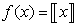is not continuous at a, isdifferentiable at a?” Next, using combined symbolic and graphical representations, he proved to the students that when x = 0, the left-hand derivatives and right-hand derivatives are not equal; therefore, f is not differentiable at 0. After generating a basic level of intuition, Dr. A continued by asking the students, “If a function f (x) is not continuous at a, is f (x) not differentiable at a? If so, then the statement ‘if a function f (x) is differentiable at a, then f (x) is continuous at a’ is true.” He then used a symbolic representation to prove the theorem in mathematical notation.

4.2. Exploration Examples

The instructional examples that were used to guide students towards particular concepts or theorems and the examples that provided evidence for the credibility of concepts or theorems that were then introduced were categorized as exploration examples. Dr. B did not use any exploration examples, whereas Dr. A used four exploration examples. The first exploration example used by Dr. A was presented prior to the introduction of Rolle’s Theorem. Dr. A first asked each student to draw a continuous, smooth curve or a straight line between the points [a,b] in their notebooks under the condition that f(a)=f(b). Next, Dr. A asked the students to connect the two endpoints (a, f (a)) and (b, f (b)) on the graph that they had just drawn. He then asked them, “Are there any tangent lines in your drawings that are special?” The students noticed either tangent lines that were parallel to the line connecting the two endpoints or that there were horizontal tangent lines. Dr. A then further asked, “How many tangent lines are there?” Due to the differences in their graphs, the students found different numbers of horizontal tangent lines, ranging from one to infinity. In the next stage, Dr. A selected several representative graphs and asked the students to draw them on the blackboard. He then guided the students in converting the graphical and verbal representations into a symbolic representation, leading to the statement of Rolle’s Theorem. Dr. A did not present a proof of Rolle’s Theorem, but rather used an exploration example to derive the theorem. The second exploration example was an extension of the first exploration example. Dr. A asked the students, “If I removed the condition ‘f(a)=f(b)’ from Rolle’s Theorem, would we get the same result?” Again, he asked each student to draw a continuous, smooth curve through points [a,b] in their notebooks. He then asked the students, “Based on what we have learned just now, what interesting phenomenon can you see in your graphs?” The students’ replies included “A tangent line parallel to the connecting line of the two endpoints can be drawn on the curve” and “Two tangent lines parallel to the connecting line of the two endpoints can be drawn on the curve.” Again, Dr. A selected several representative graphs and asked the students to draw them on the blackboard. He then guided the students in converting the graphical and verbal representations into a symbolic representation, leading to the statement of the Mean Value Theorem, and then used Rolle’s Theorem to prove the Mean Value Theorem. Students were able to observe the statement of the theorem from the exploration example, while the exploration example also provided them with “argumentation credibility.” Thus, for the students, the graphs they drew may suffice to convince them that what they saw was a definite statement uniquely determined by the nature of mathematics and is thus a credible statement. In particular, while the two exploration examples were initiated by Dr. A, they consisted of examples given by the students themselves. During the pre-observation interview, Dr. A commented that, “Students tend to memorize the theorems without understanding them. These two theorems have tremendous geometric significance. I told the students, a graph speaks louder than a thousand words. They just need to draw a graph to describe the statement of a theorem. At the same time, graphs can help them understand statements, rather than simply memorizing them. More importantly, by introducing this kind of exploration process, the students will realize that they are able to discover theorems on their own.”

4.3. Illustrating Examples

Examples that were used to exemplify an introduced theorem were categorized as illustrating examples. Illustrating examples of a theorem could be used to persuade students of the possibility of a given theorem and provide insight into the proof, rather than familiarize them with the procedural knowledge conveyed by definitions and theorems. Illustrating examples are usually presented following the description of the theorem, introduced either just before or after the proof of the theorem, and they can even serve as replacements for the proof. Dr. A and Dr. B used 8 and 13 illustrating examples of definitions and theorems, respectively. Dr. B presented his first illustrating example after he described and illustrated the definition of derivatives. He used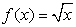as an example to calculate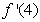. A seventh illustrating example used by Dr. B was presented after the introduction of the definition of critical numbers and the theorem, “If f has a relative extremum at c, then c is a critical number of f.” Dr. B used the graph of a complicated function at a point where the derivative equals 0 and a point where no derivatives exist to illustrate that relative extrema only occur at the critical numbers; however, he did not provide a proof of the theorem. During the pre-observation interview, Dr. B talked about why he used an illustrating example with a graph to replace the proof: “In fact, the proof of this theorem is very simple, but it involves mathematical logic; namely, the left-hand derivatives and right-hand derivatives. Since the proof of the theorem involves a complex string of symbols and ideas, the entire proof process may confuse the students.” Dr. A also agreed that the inference of the proof of the aforementioned theorem was difficult for students to master. Separately, Dr. A wanted to “use this example to help students understand the theorem and guide students to understand the proof.” Before presenting the proof, Dr. A used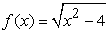as an example, and explained that the derivative of f(x) is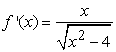. Next, Dr. A asked the students to identify the situations (the range of x) where derivatives exist, where derivatives fail to exist, where the derivative is equal to 0, and where the derivative is not equal to 0. He then used a tree graph to classify the derivatives: “There are two statuses of derivatives, existent and non-existent. When derivatives exist, the derivatives can either equal 0 or not equal 0. Thus, we can divide derivatives into three situations: derivatives fail to exist, the derivatives equal 0, and the derivatives do not equal 0. At this point, let’s go back to this theorem and read it again: if the derivative of f at c doesn’t exist, then we don’t have to prove anything; if the derivative of f at c exists, we have to prove that the derivative equals 0.”

4.4. Counterexamples

Examples that were used to illustrate that a speculation is false or to demonstrate the necessity of the conditions and hypotheses behind definitions and theorems were categorized as counterexamples. Counterexamples can also be used to provide examples that do not satisfy all of the hypotheses and then prove that the results are not true, or to provide examples that lead to altered hypotheses and altered results so as to generate a different but related theorem. Such examples are used to convey to students the credibility of the theorems or to support understanding of the concepts or theorems. Dr. A and Dr. B used six and five counterexamples, respectively. Four of the counterexamples used by Dr B. were to refute the speculation “If f is continuous at a, then f is differentiable at a.” His first counterexample used graphical and symbolic representation to show that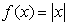is continuous when x = 0, but not differentiable. The second counterexample used graphical representation to demonstrate that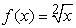is continuous when x = 0; there is a tangent line, but the tangent line has no slope; hence, it is not differentiable. The fifth counterexample illustrated a situation where a number of the hypotheses of the Mean Value Theorem are not satisfied: “Let. Examine whether the Mean Value Theorem is satisfied when f(x) is within [-1,2].” The first counterexample used by Dr. A was the same as the first counterexample used by Dr. B. His second counterexample was “If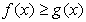,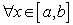, is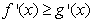,?” The third counterexample was “If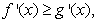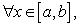is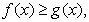?” The second and third counterexamples were presented after the introduction of the definition and calculation of derivatives. Dr. A expressed his reasons for selecting the two counterexamples in the pre-observation interview: “Firstly, I want to challenge the students’ false intuition and inspire them to think more mathematically. Secondly, the students know that a derivative is the slope of a tangent line; however, they are only able use this knowledge during calculation, rather than applying the concept to think [mathematically]. Lastly, I hope that the students can come up with their own counterexamples through discussion, rather than my telling them directly.” Initially, the students thought that both statements were correct. However, through discussion between the teacher and students, Dr. A constantly challenged the ideas of the students, providing feedback on the examples they proposed. Eventually, all students were able to give correct counterexamples.

4.5. Extending Examples

Examples that were used to extend a concept or definition after it was introduced so that the introduced concept and definition can be linked to other concepts, results, and models, were categorized as extending examples. Dr. A used three extending examples. It is worth noting that after introducing the definition of the derivative,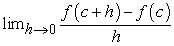, he asked the students for the definition of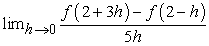. Dr. A introduced his reason for using this instructional example during the pre-observation interview: “Students tend to hold stereotypes toward the formula, based on the definition of derivatives and thoughts that they must have been given by other teachers. As a result, they are likely to have difficulties when they need to use the definition of derivatives to prove formulas or theorems, such as in the proof for the chain rule of differentiation. So, I hope that this example can help students to understand the structure of the definition of derivatives. Regardless of the appearance of the formula, as long as the structure is the same, it is a definition of a derivative.” Dr. A attempted to extend the students’ understanding of the definition of derivatives from a single symbolic representation to an expression of a structure with this example. “The numerator is the difference between the values of two functions, and the denominator is the difference between the two independent variables of the numerator. The limits of the numerator and denominator are both 0. If the denominator is not the difference between the two independent variables of the numerator, then it does not belong to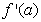.” Extending examples can be used to generate or describe examples and to define the possible variations of the category, while the variation itself falls within a range of permissible changes 13 and creates and resolves cognitive conflict 17. These observations and examples are relevant from the perspective of the theory of variation, for the comparison of variance and invariance is important to prepare students to grasp the characteristics of mathematical objects 18, 19.

### 5. Discussion and Conclusion

The types of instructional examples used by the two teachers in this study include start-up examples, exploration examples, illustrating examples, counterexamples, and extending examples. The contributions of this study include providing a better understanding of the application of corresponding examples in the teaching practice of calculus courses, as well as providing an insight into how instructors can use these examples in their calculus courses. The application of exploration and extending examples in particular has not yet been covered in previous research. The primary limitation of this study lies in the limited number of observed teachers. In addition, the instructional examples presented in this study were only a subset of the examples adopted by collegiate mathematics teachers in their calculus teaching practices. Although the research sample of the present study was small, the present researchers managed to obtain abundant examples to construct the architecture of instructional examples to teach derivatives.

Thompson identified Kuhn and Ball’s model of mathematics teaching as “constituting a consensual knowledge base regarding models of teaching” 20, 21. Kuhn and Ball’s model identifies four different teaching approaches:

A. Learner focused: mathematics teaching that focuses on the learner’s personal construction of mathematics knowledge;

B. Content-focused with an emphasis on conceptual understanding: mathematics teaching that is driven by the content itself but emphasizes conceptual understanding;

C. Content-focused with an emphasis on performance: mathematics teaching that emphasizes student performance and mastery of mathematical rules and procedures; and

D. Classroom focused: mathematics teaching based on knowledge about effective classrooms.

According to this model and based on the instructional examples used by the two teachers, it can be concluded that Dr. B’s teaching approach was more content-focused with an emphasis on performance, as he emphasized mathematical rules and procedures. According to Dr. B, the purpose of using instructional examples was to explain procedural knowledge. There was little interaction and discussion between the teacher and the students in his classes. He “automated” the calculation process and trained the students to respond to informational clues, such as specific words and contexts, in the question. During the interview, he claimed that, “if you see that the question asks you to solve the extremum, you should be able to realize that the derivatives equal 0.” Dr. A’s teaching approach was more inclined towards what Thompson described as content-focused with an emphasis on conceptual understanding 20, with its distinguishing feature, the “dual influence of content and learner. On the one hand, content is focal, but on the other, understanding is viewed as constructed by the individual” 21.

Dr. A believes that mathematics is a logically consistent knowledge-based entity that awaits discovery by students. He emphasized the understanding of concepts and encouraged students to develop their intuitive ideas towards concepts such as variability and slope. His teaching method was student-centered. He arranged classroom interactions to allow students to participate in discussions and share ideas to explore conceptual mathematical content and logic, so that each student could speculate, analyze, make decisions, and obtain their own conclusions in his calculus courses.

In general, Dr. B (content-focused with an emphasis on performance) aims towards a privileged knowledge of routine procedures and rules (using a lecture style of teaching), while Dr. A (content-focused with an emphasis on conceptual understanding) aims towards an understanding of mathematical ideas and student construction of meaning (using a student-centered style of teaching). This study provided evidence of the application of instructional examples to teach derivatives in calculus courses; however, it did not discuss the impact of these instructional examples on the students’ learning of the concepts of derivatives. Future studies are suggested to investigate the impact of various instructional examples on students’ learning of mathematical concepts.

### Acknowledgements

This article is a part of a project that is supported by the National Science Council of Taiwan (NSC 102-2511-S-131-001-MY3).

### References

  Bills, L., Dreyfuss, T., Mason, J., Tsamir, P., Watson, A. and Zaslavsky, O., “Exemplification in Mathematics Education”. In J. Novotná, H. Moraová, M. Krátká & N. Stehliková (Eds.). Proceedings of the 30th Conference of the International Group for the Psychology of Mathematics Education. Prague: Psychology of Mathematics Education, 1, 126-154, 2006. In article PubMed  Watson, A. and Mason, J., “Extending Example Spaces as a Learning/Teaching Strategy in Mathematics”. In A. Cockburn & E. Nardi (Eds.). Proceedings of the Psychology of Mathematics Education. Norwich: University of East Anglia. 2, 377-385, 2002. In article View Article  Zaslavsky, O. and Zodik, I., “Mathematics Teachers’ Choices of Examples that Potentially Support or Impede Learning”, Research in Mathematics Education, 9(1), 143-155, 2007. In article View Article  Alcock, L. and Inglis, M., “Doctoral Students’ Use of Examples in Evaluating and Proving Conjectures”, Educational Studies in Mathematics, 69, 111-129, 2008. In article View Article  Watson, A. and Chick, H., “Qualities of Examples in Learning and Teaching”, ZDM Mathematics Education, 43, 283-294, 2011. In article View Article  Harel, G. and Fuller, E., “Contributions toward Perspectives on Learning and Teaching Proof”. In D. Stylianou, M. Blanton & E. Knuth (Eds.). Teaching and Learning Proof across the Grades: A K-16 Perspective (pp. 355-370). New York, NY: Routledge, 2009. In article View Article  Speer, N., Smith, J. and Horvath, A., “Collegiate Mathematics Teaching: An Unexamined Practice”, Journal of Mathematical Behavior, 29, 99-114, 2010. In article View Article  Weber, K., “Traditional Instruction in Advanced Mathematics Courses: A Case Study of One Professor’s Lectures and Proofs in an Introductory Real Analysis Course”, Journal of Mathematical Behavior, 23, 115-133, 2004. In article View Article  Atkinson, R. K., Derry, S. J., Renkl, A. and Wortham, D., “Learning from Examples: Instructional Principles from the Worked Examples Research”, Review of Educational Research, 70(1), 181-214, 2000. In article View Article  Zaslavsky, O., “The Explanatory Power of Examples in Mathematics: Challenges for Teaching”. In M. K. Stein & L. Kucan (Eds.), Instructional Explanations in the Disciplines (pp. 107-128). Springer US, 2010. In article View Article  Zodik, I. and Zaslavsky, O., “Characteristics of Teachers’ Choice of Examples in and for the Mathematics Classroom”, Educational Studies in Mathematics, 69, 165-182, 2008. In article View Article  Zaslavsky, O. and Lavie, O., “Teachers’ Use of Instructional Examples”. Paper presented at the 15th Study Conference of the International Commission on Mathematical Instruction (ICMI), on the Professional Education and Development of Teachers of Mathematics. Águas de Lindóia, Brazil, 2005. In article  Leinhardt, G., Zaslavsky, O. and Stein, M. K., “Functions, Graphs, and Graphing: Tasks, Learning, and Teaching”, Review of Educational Research, 60(1), 1-64, 1990. In article View Article  Goldenberg, P. and Mason, J., “Shedding Light on and with Example Space”, Educational Studies in Mathematics, 69, 183-194, 2008. In article View Article  Michener, E. R., “Understanding Understanding Mathematics”, Cognitive Science, 2, 361-383, 1978. In article View Article  Glaser, B. and Strauss, A., The Discovery of Grounded Theory: Strategies for Qualitative Research. New Brunswick, NJ: Aldine Transaction, 1967. In article PubMed  Zazkis, R. and Chernoff, E. J., “What Makes a Counterexample Exemplary?”, Educational Studies in Mathematics, 68, 195-208, 2008. In article View Article  Marton, F. and Booth, S., Learning and Awareness. Mahwah, NJ: Lawrence Erlbaum, 1997. In article View Article  Marton, F. and Pang, M. F., “Two Faces of Variation”, Paper presented at the 8th European Conferences for Learning and Instruction, August 24-28, University of Göteborg, Sweden, 1999. In article View Article  Thompson, A. G., “Teachers’ Beliefs and Conceptions: A Synthesis of the Research”. In D. A. Grouws (Ed.), Handbook of Research on Mathematics Teaching and Learning (pp. 127-146). New York, NY: Macmillan, 1992. In article View Article  Kuhn, T. and Ball, D., Approaches to Teaching Mathematics: Mapping the Domains of Knowledge, Skills and Dispositions. East Lansing, MI: Michigan State University, Center on Teacher Education, 1986. In article

Published with license by Science and Education Publishing, Copyright © 2017 Chih-Hsien HuangThis work is licensed under a Creative Commons Attribution 4.0 International License. To view a copy of this license, visit http://creativecommons.org/licenses/by/4.0/

### Cite this article:

##### Normal Style
Chih-Hsien Huang. Teachers’ Choice and Use of Examples in Teaching Derivatives. American Journal of Educational Research. Vol. 5, No. 11, 2017, pp 1152-1157. http://pubs.sciepub.com/education/5/11/6
##### MLA Style
Huang, Chih-Hsien. "Teachers’ Choice and Use of Examples in Teaching Derivatives." American Journal of Educational Research 5.11 (2017): 1152-1157.
##### APA Style
Huang, C. (2017). Teachers’ Choice and Use of Examples in Teaching Derivatives. American Journal of Educational Research, 5(11), 1152-1157.
##### Chicago Style
Huang, Chih-Hsien. "Teachers’ Choice and Use of Examples in Teaching Derivatives." American Journal of Educational Research 5, no. 11 (2017): 1152-1157.